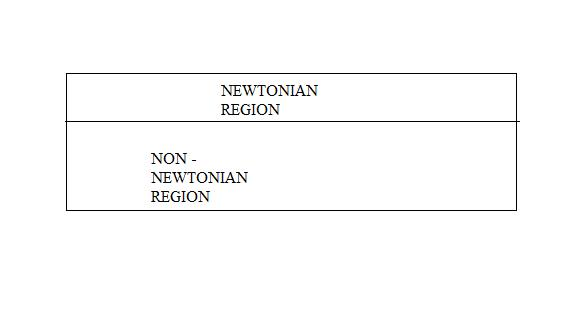## Fluids

•DEBA
Subscriber

SIR,

I HAVE  DRAWN  DIFFERENT REGION IN MY GEOMETRY IN ICEM CFD. I HAVE ALSO WRITTEN UDF CODE FOR THE PROPERTY OF NON NEWTONIAN REGION AND PROPERTY RELATED TO NEWTONIAN REGION  KNOWN TO ME. MY QUERY TO YOU SIR

1) HOW I WILL ASSIGN DIFFERENT PROPERTY IN DIFFERENT ZONE THAT IS UDF CODE FOR NON NEWTONIAN & KNOWN PROPERTY TO NEWTONIAN.

2) I AM UNABLE TO DO  DIFFERENT PROPERTY FOR DIFFERENT ZONES IN FLUENT AFTER IMPORT THE MESH FILE FROM ICEM CFD

3) PERPHERI IS NEWTONIAN AND ANOTHER TWO REGION IS NON NEWTONIAN.•DrAmine
Ansys Employee

What do you mean with non-Newtonian region? Do you two fluids in your problem with different viscosity laws? Or do you have only one fluid but the viscosity law would depend on the distance from the axis or wall or whatever?

•DEBA
Subscriber

Fluid is blood . Near the center line it is treated by one viscosity(non-newtonian)  law and near the wall it is treated as another viscosity (newtonian) law

•DrAmine
Ansys Employee

Okay. I won't discuss the technical part but only the feasibility.  You can model that by using the wall distance as the dependent variable and make a blending between the viscosity laws based on the cell wall distance. You will require some UDF's to do that.

•Rob
Ansys Employee

Hi,  you might want to read

https://www.ncbi.nlm.nih.gov/pmc/articles/PMC1552100/   and

Gijsen F.J.H., van de Vosse F.N., Janssen J.D.; 1999; The Influence of the non-Newtonian properties of blood on the flow in large arteries: steady flow in a carotid bifurcation model; Jou of Biomechanics, Vol 32, pp601

We used the latter in several projects during the mid-00s when I was looking at MRI conversion work. A colleague used the Casson model, based around

Fournier, Basic Trans Phenom in Biomed Eng, p. 73

•DEBA
Subscriber

I HAVE WRITTEN VISCOSITY UDF FOR CASSON

•DrAmine
Ansys Employee

Okay so you can apply it as it would respond dynamically to strain.

•Karthik R

Hello,

As put by both abenhadj and rwoolhou earlier, the idea here is to choose a non-Newtonian model whose response is

• similar to a Newtonian fluid near the wall, and

• similar to a non-Newtonian fluid near the center

To do not explicitly need two fluid domains to model this.

Several models of blood are available in literature which might show this behavior. You might have to find a model which best showcases this and use that as a UDF. However, before running a non-Newtonian model, I'd strongly recommend you test your model set-up using a newtonian fluid and make sure that you are getting the desired convergence. Once you make sure that your results are physical, you can then attempt to model a non-Newtonian fluid.

Good luck!

Best Regards,

Karthik

•DEBA
Subscriber

sir i GOT converge result both i newtonian and non newtonian model.

I want to write udf of viscosity as a function distance from center

Below i have attached picture.

for radius 0 to 30 meter viscocity is a (let)

for radius 30 to 50 viscocity is b•DEBA
Subscriber

sir i GOT converge result both i newtonian and non newtonian model.

I want to write udf of viscosity as a function distance from center

Below i have attached picture.

for radius 0 to 30 meter viscocity is a (let)

for radius 30 to 50 viscocity is b•Karthik R

Hello,

Since you have created a new post, I have left my reply there. I hope this helps.

Best Regards,

Karthik

•DrAmine
Ansys Employee

Hi,

Please go through all suggestions here related to applying Casson model or a non-Newtonian model in general as that would fit your case. This has been highlighted by my colleagues and myself so far.

If you want however to incorporate the wall distance in your viscosity formulation you need:

1/Get wall distance in laminar case: wall distance is not calculated for laminar. Here you need apply Poisson equation to get the wall distance or work with turbulence model but set the zone to laminar.

2/Use the stored wall distance and use it in a DEFINE_PROPERTY UDF to define the viscosity.

•DEBA
Subscriber
•DrAmine
Ansys Employee